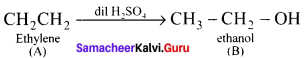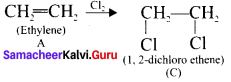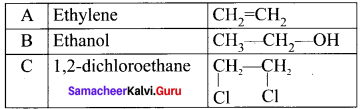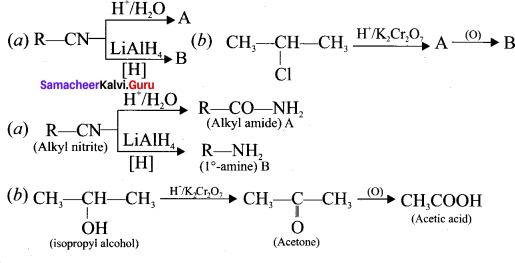# Samacheer Kalvi 11th Chemistry Solutions Chapter 12 Basic Concepts of Organic Reactions

The Samacheer Kalvi Class 11th Chemistry Solutions Chapter 12 Basic Concepts of Organic Reactions Questions and Answers prevailing here are designed by the academic subject expertise in accordance with the state board prescribed syllabus and books. Aspirants who require help in preparing the Tamilnadu State Board Class 11th Chemistry Solutions Chapter 12 Basic Concepts of Organic Reactions exercise questions can rely on the Samacheer Kalvi 11th Chemistry Solutions pdf for Chapter 12 Basic Concepts of Organic Reactions given in this article.

## Tamilnadu Samacheer Kalvi 11th Chemistry Solutions Chapter 12 Basic Concepts of Organic Reactions

Students who are excited for Tamilnadu State Board for Class 11th Chemistry Solutions Chapter 12 Basic Concepts of Organic Reactions can find detailed and step wise solutions for all questions of Chapter 12 Basic Concepts of Organic Reactions from here. Simply tap on the links available over here for which you have to view/download the Samacheer Kalvi Class 11th Solutions for Chemistry Solutions chapter 12 Basic Concepts of Organic Reactions Questions and Answers. All these solutions are free to download and easy to access online or offline so that you can prepare well for the exams at any time.

### Samacheer Kalvi 11th Chemistry Basic Concepts of Organic Reactions Textual Evaluation Solved

Question 1.
For the Following reactions –
(A) CH3 CH2 CH2 Br + KOH → CH3 – CH + KBr + H2O
(B) (CH3 )3 CBr + KOH → (CH3 )3 COH + KBr
(C)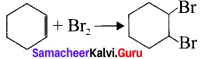Which of the following statement is correct’?
(a) (A) is elimination, (B) and (C) are substitution
(b) (A) is substitution, (B) and (C) are elimination
(c) (A) and (B) are elimination and (C) is addition reaction
(d) (A) is elimination, B is substitution and (C) is addition reaction.
(d) (A) is elimination, B is substitution and (C) is addition reaction.

Question 2.
What is the hybridisation state of benzyl carbonium ion?
(a) sp2
(b) spd2
(c) sp3
(d) sp2d
(a) sp2

Question 3.
Decreasing order of nucleophilicity is –
(a) OH> RNH2 > OCH3> RNH2
(b) NH2> OH> OCH3> RNH2
(c) NH,> CH3O >OH> RNH2
(d) CH3O> NH2> OH> RNH2
(b) NH2> OH> OCH3> RNH2+

Question 4.
Which of the tòllowing species is not eLectrophilic in nature?
(a) Cl+
(b) BH3
(c) H3O+
(d) +NO2
(c) H3O+

Question 5.
Homolytic fission of covalent bond leads to the formation of –
(a) electrophile
(b) nucicophile
(c) Carbocation

Question 6.
Hyper Conjugation is also known as –
(a) no bond resonance
(b) Baker – nathan effect
(c) both (a) and (b)
(d) none of these
(c) both (a) and (b)

Question 7.
Which of the group has highest +I effect?
(a) CH3
(b) CH3CH2
(c) (CH3)2 -CH-
(d) (CH3)3-C-
(d) (CH3)3-C-

Question 8.
Which of the following species does not exert a resonance effect?
(a) C6H5OH
(b) C6H5Cl
(c) C6H5NH2
(d) C6H5NH3
(d) C6H5NH3

Question 9.
-I effect is shown by –
(a) -Cl
(b) -Br
(c) both (a) and (b)
(d) -CH3
(c) both (a) and (b)

Question 10.
Which of the following carbocation will be most stable?
(a) $${ PH }_{ 3 }\overset { + }{ C- }$$
(b) CH3–$$\overset { + }{ C{ H }_{ 2 } }$$
(c) (CH3)2–$$\overset { + }{ C{ H } }$$
(d) CH2 = CH-CH3
(a) $${ PH }_{ 3 }\overset { + }{ C- }$$

Question 11.
Assertion : Tertiary Carbocations are generally formed more easily than primary Carbocations.
Reason : Hyper conjugation as well as inductive effect due to additional alkyl group stabilize tertiary carbonium ions.
(a) both assertion and reason arc true and reason is the correct explanation of assertion.
(b) both assertion and reason are true but reason is not the correct explanation of assertion.
(c) Assertion is true but reason is false
(d) Both assertion and reason are false
(a) both assertion and reason are true and reason is the correct explanation of assertion.

Question 12.
Heterolytic fission of C-C bond results in the formation of –
(b) Carbanion
(c) Carbocation
(d) Carbanion and Carbocation
(e) Carbocation

Question 13.
Which of the following represent a set of nucleophiles?
(a) BF3, H2O, NH2-
(b) AlCl3, BF3, NH3
(c) CN, RCH2, ROH
(d) H+, RNH+2, CCl2
(c) CN, RCH2, ROH

Question 14.
Which of the following species does not acts as a nucleophile?
(a) ROH
(b) ROR
(c) PCl3
(d) BF3
(d) BF3

Question 15.
The geometrical shape of carbocation is
(a) Linear
(b) tetrahedral
(c) Planar
(d) Pyramidal
(c) Planar

II. Write brief answer to the following questions.

Question 16.
Write short notes on:
(a) Resonance
(b) Hyper conjugation
(a) Resonance:
1. Certain organic compounds can be represented by more than one structure and they differ only in the position of bonding and lone pair of electrons. Such structures are called resonance structure and this phenomenon is called as resonance. This phenomenon is also called as mesomerism or mesomeric effect.

2. For example, the structure of aromatic compounds such as benzene and conjugated system like 1,3 butadiene cannot be represent by a single structure and their observed properties can be explained on the base of a resonance hybrid.

3. Resonance structure of benzene.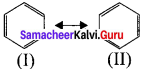(I) and (II) are called as resonance hybridš of benzcnc.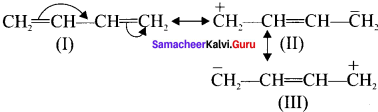(I), (II) and (III) are called as resonance hybrids of 1,3 butadiene.

(b) Hyper conjugation:
1. The denationalization of electrons of σ bond is called as hyper conjugation. it is a special stabilizing effect that results due to the interaction of electrons of a σ bond with the adjacent empty non-bonding P-orbitais resulting in an extended molecular orbital.

2. Hyper conjugation is a permanent effect.

3. For example, in propane. the σ- electrons of C-H bond of methyl group can be delocalised into the it- orbital of doubly bonded carbon as represented below.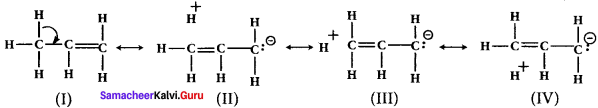Question 17.
What are electrophiles and nucleophiles? Give suitable examples for each.
Electrophiles:
Electrophiles are reagents that are attracted towards negative charge or electron rich center. They are either positively charged ions or electron deficient neutral molecules.
Example:
CO2, AlCl3, BF3, FeCl3, NO+, NO+2,, etc.

Nucleophiles:
Nucleophiles are reagents that has high affinity for electropositive centers. They possess an atom has a unshared pair of electrons. They are usually negatively charged ions or electron rich neutral molecules.
Example.
NH3, R-NH2, R-SH, H2O, R-OH, CN. OH etc.

Question 18.
Show the heterolysis of covalent bond by using curved arrow notation and complete the following equations. Identify the nucleophile In each case.
1. CH3-Br+KOH →
2. CH3-OCH3+HI →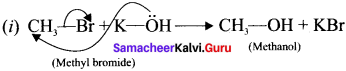In this case, $$\overset { \ominus }{ Br }$$ and $$\overset { \ominus }{ OH }$$ are nucleophiles.

Another mechanism:
Step – I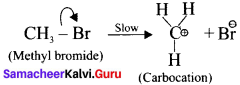Step-II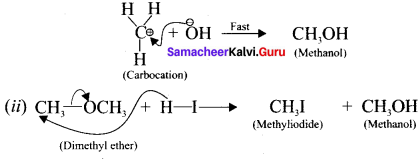In this case, OCH3 and -I are nucleophiles
Another mechanism:
Step – I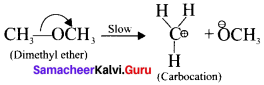Step – II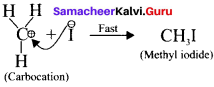Question 19.
Explain inductive effect with suitable example.
Inductive effect:
1. it is defined as the change ¡n the polarization of a covalent bond due to the presence of adjacent bonded atoms or groups in the molecule. It is denoted as I-effect.

2. Atoms or groups which lose electron towards a carbon atom are said to have a +I effect.
Example:
CH3-,(CH3)2 CH-,(CH3)2 C- etc.

3. Atoms or groups which draw electrons away from a carbon atom are said to have a -I effect.
Example:
-NO2. -I, -Br, -OH, C6H5 etc.

4. For example, consider ethane and ethyl chloride. The C-C bond in ethane is non polar while the C-C bond in ethyl chloride is polar. We know that chlorine is more electronegative than carbon and hence it attracts the shared pair of electrons between C-Cl in ethyl chloride towards it self.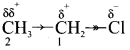This develops a slight negative charge on chlorine and a slight positive charge on carbon to which chlorine is attached. To compensate it, the C1 draws the shared pair of electron between itself and C2. This polarization effect is called inductive effect.

Question 20.
Explain electromeric effect.
Electromeric effect:
1. The electromeric effect refers to the polarity produced in a multiple bonded the compound when it is attacked by a reagent when a double or a triple bond is exposed to an attack by an electrophile the two π electrons which from the π bond are completely transferred to one atom or the other.

2. When a nucleophile approaches the carbonyl compound, the π-electrons between C and O is instantaneously shifted to the more electronegative oxygen. This make the carbon electron deficient and thus facilitating the formation of a new bond between the incoming nucleophile and the carbonyl carbon atom.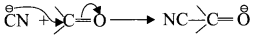3. When an electrophile such as H approaches an alkene molecule their electrons are instantaneously shifted to the electrophiie and a new bond is formed between carbon and hydrogen. This makes the other carbon electron deficient and hence it acquires a positive charge.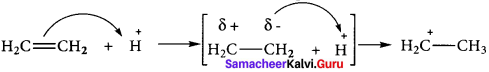4. This effect denotes as E-effect.

Question 21.
Give examples for the following types of organic reactions
1. – elimination
2. Electrophilic substitution.
1. β-eIimination:
Elimination reactions involve the cleavage of a a bond and tórmation of air bond. A nucleophilic pair of electrons heads into a new it bond as a leaving group departs. This process is called 3-elimination because the bond Í to the nucleophilic pair of electrons breaks.
Example:
(a) n-propyl bromide on reaction with alcoholic KOH give propene. in this reaction hydrogen and Br are eliminated.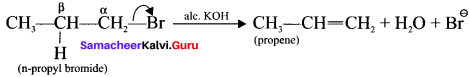(b) Acid-catalysed dehydration of alcohols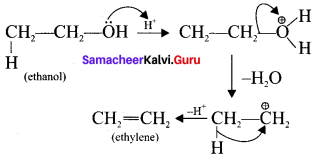2. Electrophilic substitution:
Substitution reactions when are brought about by electrophiles are called electrophilic substitution reaction.
Example:
(a) Nitration of benzene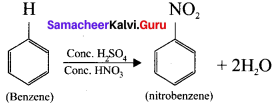(b) Bromination of benzene: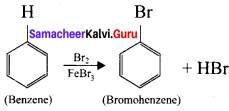### Samacheer Kalvi 11th Chemistry Basic Concepts of Organic ReactionsAdditional Questions Solved

Question 1.
Statement-I: All the organic molecules contain covalent bonds.
Statement-II: Organic molecules are formed by the mutual sharing of electrons between atoms.
(a) Statement-I and II are correct and statement-II is correct explanation of statement-I.
(b) Statement-I and II are correct but Statement-II is not correct explanation of statement-I.
(c) Statement-I is correct but statement-II is wrong.
(d) Statement-I is wrong but statement-II is correct.
(a) Statement-I and II are correct and statement-II is correct explanation of statement-I.

Question 2.
Statement-I : Homolytic cleavage is symmetrical one.
Statement-II : A single covalent bond breaks and each of the bonded atoms retains one electron.
(a) Statement-I and II are correct and statement-II is correct explanation of statement-I.
(b) Statement-I and II are correct but statement-II is not correct explanation of statement-I.
(c) Statement-I is correct but statement-II is wrong.
(d) Statement-I is wrong but statement-II is correct.
(a) Statement-I and II are correct and statement-Il is correct explanation of statement-I.

Question 3.
(a) Benzoyl peroxide
(b) Benzyl alcohol
(c) Benzyl acetate
(d) Benzaldehyde.
(a) Benzoyl peroxide.

Question 4.
Which one of the following ¡s correct order of stability of alkyl free radicals?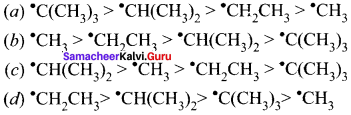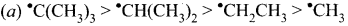Question 5.
Statement-I: Ileterolytic cleavage is unsymmetrical one.
Statement-II: A covalent bond breaks and one of the bonded atom retains the bond pair of electrons.
(a) Statement-I and II are correct and statement-II is correct explanation of statement-I.
(b) Statement-I and II are correct but statement-II is not correct explanation of statement-I.
(c) Statement-I is correct but statement-II is wrong.
(d) Statement-I is wrong but statement-II is correct
(a) Statement-I and II are correct and statement-II is correct explanation of statement-I.

Question 6.
Which of the following is correct order of the stability of carbocations?
(a) +CH3 > +CH2 CH3> +CH(CH3)2 > +C(CH3)3
(b) +CH2CH3 > +CH3 >+ CH(CH3)2 > +C(CH3)3
(c) +C(CH3)3 > +CH(CH3)2 >+CH2CH3CH3
(d) +CH(CH3)2 > +CH3 > +CH2CH3 > +C(CH3)3
(c) +C(CH3)3 > +CH(CH3)2 >+CH2CH3CH3

Question 7.
Which one of the following is correct order of the stability of carbanions?
(a) C(CH3)3> CH(CH3)2> CH2-CH3> CH3
(b) CH3> CH2-CH3> CH(CH3)2> C(CH3)3
(c) CH(CH3), > CH3> CH2-CH3 > C(CH3)3
(d) CH2-CH3 > CH(CH3)2> CH3> C(CH3)3
(b) CH3> CH2-CH3> CH(CH3)2> C(CH3)3

Question 8.
Which one of the following is not electrophile’?
(a) NH3
(b) AlCl3
(c) FeCl3
(d) R-X
(a) NH3

Question 9.
Which one of the following is not nucleophile
(a) H2O
(b) NH3
(c) R-OH
(d) FeCl3
(d) FeCl3Question 10.
Which one of the following is positively charged electrophiles?
(a) CO2
(b) AlCl3
(c) BF3
(d) RX
(d) RX

Question 11.
Which one of the following is nucleophile?
(a) BF3
(b) AlCl3
(c) CO2
(d) R-SH
(d) R-SH

Question 12.
Which one of the following species has tendency to show-I effect?
(a) -CH3
(b) -CH2-CH3
(c) -CH(CH3)2
(d) -C6H5
(d) -C6H5

Question 13.
Which one of the following species has tendency to show +I effect?
(a) -NH2
(b) -Cl
(c) -C6H5
(d) -CH3
(d) -CH3

Question 14.
Which one of the following has strongest acidic character?
(a) HCOOH
(b) CH3COOH
(c) CH2ClCOOH
(d) CCl3COOH
(d) CCl3COOH

Question 15.
Which one of the following has least acidic character?
(a) HCOOH
(b) CH3COOH
(c) CH2ClCOOH
(d) CCl3COOH
(b) CH3COOH

Question 16.
Pick out the correct order of acid strength.
(a) CH3-CH2-COOH > CH3COOH > CH2CICOOH
(b) CH3COOH > CH3-CH2-COOH >CH2CICOOH
(c) CH2ClCOOH > CH3COOH > CH3-CH2-COOH
(d) CH2ClCOOH > CH3-CH2-COOH > CH3COOH
(c) CH2ClCOOH > CH3COOH > CH3—CH2-COOH

Question 17.
Statement-I: Fluoro acetic acid is stronger acid than acetic acid
Statement-II: Fluorine has high electronegativity and it is facilitate to dissociate the OH bond easily.
(a) Statement-I and II are correct and statement-II is correct explanation of statement-I.
(b) Statement-I and II are correct but statement-II is not correct explanation of statement-I.
(c) Statement-I is correct but statement-II is wrong.
(d) Statement-I is wrong but statement-Il is correct
(a) Statement-I and II are correct and statement-II is correct explanation of statement-I.

Question 18.
Which one of the following is an example for negative mesomeric effect?
(a) -SH
(b) -SR
(c) -NH2
(d) -NO2
(d) -NO2

Question 19.
Which one of the following electrophile used for nitration of benzene?
(a) $${ Br }^{ \oplus }$$
(b) $${ { NO }_{ 2 }^{ \ominus } }$$
(c) -NH2
(d) $${ NO }^{ \ominus }$$
(b) $${ { NO }_{ 2 }^{ \ominus } }$$

Question 20.
Identify the one which does not come under the organic addition reaction
(a) Hydration
(b) Dehydration
(c) Halogenation
(d) Hydro halogenation
(b) Dehydration

Question 21.
Primary alcohols undergo which type of reaction to form alkenes?
(a) Elimination
(b) Oxidation
(c) Reduction
(d) Hydrolysis
(a) Elimination

Question 22.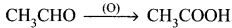Identify the type of reaction
(b) Elimination reaction
(c) Reduction reaction
(d) Oxidation reaction
(d) Oxidation reaction

II. Match the following.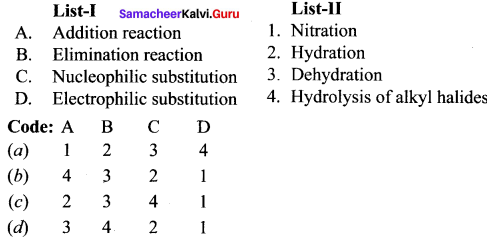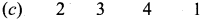III. Fill in the blanks.
Question 1.
The slowest step in the mechanism determines ………..
Rate of reaction

Question 2.
Homolytic cleavage occurs under the conditions of ………….
High temperature.

Question 3.
During the cleavage of Azobisisobutyronitrile produces species ……….

Question 4.
The cleavage of C-Br bond in tert-butyl bromide leads to formation of ………..
Carbocation

Question 5.
The cleavage of C-H bond in aldehydes Leads to formation of ………….
Carbanion

Question 6.
Electron displacement occurring in saturated compounds along a carbon chain is termed as …………
Inductive effect

Question 7.
The addition of H to alkene is an example of effect ……….
+E

Question 8.
Example for positive mesomeric effect is ………..
-OH

Question 9.
Acidity of phenol was explained by …………
R-effect

Question 10.
Hydrolysis of alkyl halide is an example for ……….
Nucleophilic substitution

Question 11.
4-hydroxy phenol reacts with acidified potassium dichromate to gives
p-Benzoquinone

Question 12.
Enzyme present in apple is
Poly phenol oxidase

Question 13.
Benzene reacts with H2 in the presence of Pt to give
Cyclohexane

Question 14.
Alcohol on refluxing with K2Cr2O7 gives……..
Carboxylic acid

Question 15.
Carbonyl compounds especially ketones undergo reduction to form ………..
Secondary alcohols

Question 16.
Ethane undergo thrombolytic cleavage to form ………..

IV. Choose the odd one out.
Question 1.
(a) NH3
(b) H2O
(c) CN
(d) RSH
(c) CN It is a negatively charged nucleophile whereas others are neutral nucleophiles.

Question 2.
(a) CO2
(b) RX
(c) MCl3
(d) FeCl3
(b) RX. It is a positive charged electrophiles whereas others are neutral clectrophiles.

Question 3.
(a) -F
(b) – Cl
(c) – COOH
(d) CH3O
(d) CH3O. It is a group carrying negative charge one electron donating (or) +I group whereas others are -I group.

Question 4.
(a) – C(CH3)3
(b) – COOK
(c) – CH(CH3)2
(d) – COO
(b) – COOH. It has -I effect whereas other have +I effect.

Question 5.
(a) -OH
(b) -NH2
(c) -SH
(d) -COOH
(d) – COOH. It has negative mesomeric effect whereas others have positive mesomeric effect.

V. Choose the correct pair.
Question 1.
(a) Homolytic clevage : carbocation
(b) Homolytic clevage : carbanion
(c) Homolytic clevage : free radicals
(d) Heterolytic clevage : free radicals
(c) Homolytic clevage : free radicals

Question 2.
(a) + C(CH3)3 > +CH(CH3)2> +CH2-CH3 > +CH3 : Relative stability of carbocations.
(b) – C(CH3)3 > CH(CH3)2 > CH2-CH3 > CH3 : Relative stability of carbanion.
(c) OH,RO, RCOO : Neutral nucleophile
(d) AlCl3, BF3, FeCl3 : Positively charged nucleophile
(a) + C(CH3)3 > +CH(CH3)2> +CH2-CH3 > +CH3 : Relative stability of carbocations.

Question 3.
(a) CH3 – CH2 – CH2Br + Alcoholic KOH : Substitution reaction
(b) CH3 – CH2 – CH2Br + Alcoholic KOH : Elimination reaction
(c) CH3CHO + Acidic dichromate : Reduction reaction
(d) Benzene + Pt + H2 : Oxidation reaction
(b) CH3 – CH2 – CH2Br + Alcoholic KOH : Elimination reaction

VI. Choose the incorrect pair.
Question 1.
(a) – OH,- SH, -NH2 : Positive mesometic effect
(b) – NO2 >CO, – COOH : Negative mesomeric effect
(c) – F, – Cl, – NO2 : Electron withdrawing group
(d) – C(CH3)3, – CH(CH3)3 – CH2 – CH3 : Electron withdrawing group
(c) – F, – Cl, – NO2 : Electron withdrawing group

Question 2.
(a) NH3 andAmines : Neutral nucleophile
(b) OH and RCOO : Negative nucleophile
(c) RX and H3O3 : Positive electrophile
(d) MCl3, BF3 : Negative electrophile
(d) AlCl3, BF3 : Negative electrophile

Question 3.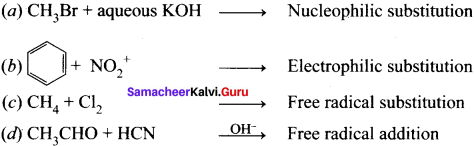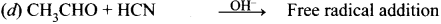VII. Assertion and Reason.
Question 1.
Assertion (A) : Neutral molecule SnCl4 can act as an electrophile.
Reason (R) : it has vaccant ‘d’ orbitais which can accommadate the electrons from others.
(a) Both (A) and (R) are correct and (R) is the correct explanation of (A).
(b) Both (A) and (R) are correct but (R) is not the correct explanation of (A).
(c) (A) is correct but (R) is wrong.
(d) (A) is wrong but (R) is correct.
(a) Both (A) and (R) are correct and (R) is the correct explanation of (A).

Question 2.
Assertion (A) : The C-C bond in ethane is non-polar while the C-C bond in ethyl chloride is polar.
Reason (R) : Chlorine is more electronegative than carbon and hence it attracts the shared pair of electron between C-C in ethyl chloride and it develops a negative charge on Cl and positive charge on Carbon.
(a) Both (A) and (R) are correct but (R) is not the correct explanation of (A).
(b) Both (A) and (R) are correct and (R) is the correct explanation of (A).
(c) (A) is correct but (R) is wrong.
(d) (A) is wrong but (R) is correct.
(b) Both (A) and (R) are correct and (R) is the correct explanation of (A).

Question 3.
Assertion (A): Phenol is more acidic than aliphatic alcohols.
Reason (R): The phenoxide ion is more stabilized than phenol by resonance effect and hence resonance favours ionization of phenol to form H and shows acidity.
(a) Both (A) and (R) are correct and (R) is the correct explanation of(A).
(b) Both (A) and (R) are correct but (R) is not the correct explanation of(A).
(c) (A) is correct but (R) is wrong.
(d) (A) is wrong but (R) is correct.
(a) Both (A) and (R) are correct and (R) is the correct explanation of(A).

Question 4.
Assertion (A): The cut apple turns brown.
Reason (R): Cut apple exposes its cells to atmospheric oxygen and the oxidizes the phenolic compound present in it. Due to this enzymatic browning, the cut apple turns brown.
(a) Both (A) and (R) are correct but (R) is not the correct explanation of (A).
(b) Both (A) and (R) are correct and (R) is the correct explanation of (A).
(c) (A) is correct but (R) is wrong.
(d) (A) is wrong but (R) is correct.
(b) Both (A) and (R) are correct and (R) is the correct explanation of (A).

Samacheer Kalvi 11th Chemistry Basic Concepts of Organic Reactions 2 Marks Question and Answers

Question 1.
What are organic reactions?
Substrate is an organic molecule reacts with reagent, which may be an organic, inorganic or any agent like heat, photon etc, that brings about the chemical change to form a product, this is known as organic reactions.

Question 2.
What is mechanism of the reaction?
In the organic reactions, that series of simple steps which collectively represent the chemical change, from substrate to product, this is called as the mechanism of the reaction. The slowest step in the mechanism determines the overall rate of the reaction.

Question 3.
Mention the types of fission of a covalent bond?
There are three types of fission of a covalent bond. They are,

• Homolytic cleavage
• Heterolytic cleavage

Question 4.
Explain the homolytic fission of a covalent bond?

• Homolytic cleavage is the process in which a covalent bond breaks symmetrically in such way that each of the bonded atoms retains one electron.
• This type of cleavage occurs under high temperature or in the presence of UV light.
• in a compound containing non-polar covalent bond formed between atoms of similar electronegativity, in such molecules the cleavage of bonds results into free radicals.
• For example, ethane undergo homolytic fission to produce, two methyl free radicals.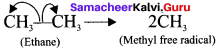Question 5.
The types of reagents that promote homolytic cleavage in substrate are called as free radical initiators. They are short lived and are highly reactive.

Question 6.

• Azobisisobutyronitnie (AIBN)
• Benzoyl peroxide

Question 7.
Explain the heterolytic fission of a covalent bond?

• Heterolytic cleavage is the process in which a covalent bond breaks unsymmetrically such that one of the bonded atom retains the bond pair of electron.
• It results in the formation of a cation and an anion of the two bonded atoms the most electronegative.
• For example in tert-butyibromide, the C-Br bond is polar as bromine is more electronegative than carbon. Hence the C-Br undergoes heterolytic cleavage to form a terl-butyl carbocation and bromide anion.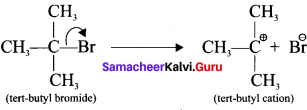Question 8.
What are carbocations?
1. Let us consider the heterolytic fission of the bond C-X present in organic molecule. If the atom X has greater electronegativity than the carbon atom, the former takes away the bonding electron pair and becomes negatively charged while the carbon will lose its electron and thus acquire a positive charge.
2. Such a cationic species carrying a positive charge on carbon are known as carbocation or carhonitim ion.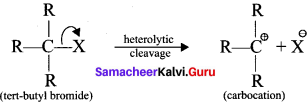Question 9.
What are carbanions?
Let us consider the heterolytic fission of the bond C-X present in an organic molecule. if the carbon atom has greater electronegativity than the atom X, the former takes away the bonding electron pair and acquires a negative charge. The resulting carbon anion is known as carbanion.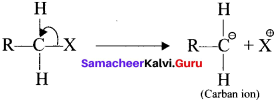Question 10.
Differentiate the carbocation and carbanion
Carbocation:

• In a carbocation carbon bearing positive charge.
• Carbon bearing positive charge has sp2 hybridization.
• It has a planar structure.
• Example:
CH3+ (CH3)2$${ C }^{ \ominus }$$H, (CH3)3C+ etc.

Carbanion:

• In a carbanion carbon bearing negative charge.
• Carbon bearing negative charge has sp3 hybridization.
• It has a pyramidal structure.
• Example:
CH3–$${ C }^{ \ominus }$$H2, CH3-CHO, (CH3)2$${ C }^{ \ominus }$$H etc.

Question 11.
Identify which of the following are electrophiles and nucleophiles?

1. NH3
2. AlCl3
3. R-SH
4. R-X
5. R-O-R
6. BF3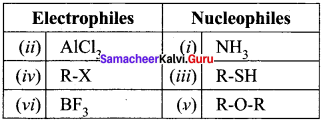Question 12.
How will you distinguish between electrophiles and nucleophiles?
Electrophiles:

• They are electron deficient.
• They are cations.
• They are lewis acids.
• Accept an electron pair.
• Attack on electron rich sites.

Nucleophites:

• They are electron rich.
• They are anions.
• They are lewis bases.
• Donate an electron pair.
• Attack on electron deficient sites.

Question 13.
What are all the sources for human body that produces free radicals?
Sources for human body produces free radicals,

• Human body is exposed to X-rays.
• Cigarette smoke.
• Industrial chemicals.
• Air pollutants.

Question 14.
In what way free radical affect the human body?

• Free radicals can disrupt cell membranes.
• Increase the risk of many forms of cancer.
• Damage the interior lining of blood vessels.
• leads to a high risk of heart disease and stroke.

Question 15.
How to reduce the effect of free radicals?

• Use vitamins and minerals to counter the effects of free radicals.
• Fruits contains antioxidants which decrease the effects of free radicals.

Question 16.
Identify which of the following shows +I and -I effect?

1. -NO2
2. -SO2H
3. -I
4. -OH
5. CH2O-
6. CH2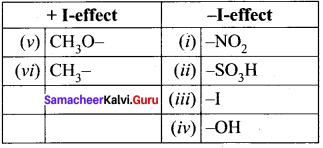Question 17.
Why chloro acetic acid is stronger acid than acetic acid?
Chioro acetic acid: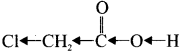Chioro acetic acid has Cl-group and it has high electronegativity and shows -I effect. Therefore Cl-atom to faciLitate the dissociation of O-H bond very fastly, whereas in the case of acetic acid, has CH3 group and it shows +I effect, theretòre dissociation of O-H bond will be more difficult. Thus chloro acetic acid is stronger acid than acetic acid.

Question 18.
Explain the positive and negative electromeric effects?
1. When an electrophile such as W approaches an alkene molecule, the π electrons are instantaneously shifted to the electrophile and a new bond is formed between carbon and hydrogen. This makes the other carbon electron deficient and hence it acquires a positive charge.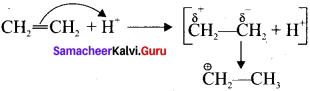2. When the it electron is transferred towards the attacking reagent, it is called positive electromeric (+E) effect.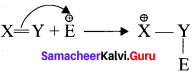3. Whèn the t electron is transferred away from the attacking reagent it is called negative electromenc (-E) effect.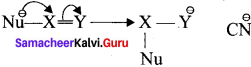For example : The attack of C$${ N }^{ \ominus }$$ on a carbonyl carbon.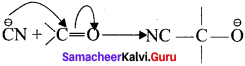Question 19.
Write a short notes on positive mesomeric effect?

• Positive mesomeric effect occurs, when the electrons move away from substituent attached
to the conjugated system.
• It occurs, if the electron releasing substituents are attached to the conjugated system.
• The attached group has a tendency to release electrons through resonance, these electron
releasing groups are usually denoted as +R or +M groups
• Examples: -OH, -SH, -OR, -SR, -NH2 etc.

Question 20.
Write a short notes on negative mesomeric effect?

• Negative mesomeric effect occurs, when the electrons move towards the substituent attached to the conjugated system.
• It occurs if the electron withdrawing substituents are attached to the conjugated system.
The attached group has a tendency to withdraw electrons through resonance, these electron withdrawing groups are usually denoted as -R or -M groups.
• Examples: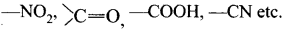Question 21.
What are addition reactions? Give an example.
All organic compounds having double or triple bond adopt addition reactions in which two substances unite to form a single compound. During the addition reaction the hybridization of the substrate changes as only one bond breaks and two new bonds are formed.
Example:Question 22.
What are elimination reaction? Give an example.
In these reactions, two atoms or groups are removed from a molecule without being substituted by other atoms or groups. A new C-C double bond is formed between the carbon atoms to which the eliminated atoms or groups are previously attached. It is always accompanied with change in hybridization.
Example: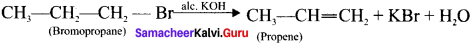Question 23.
What are organic oxidation reactions? Give an example.
Most of the oxidation reaction of organic compounds involves gain of oxygen or loss of hydrogen.
Example: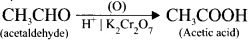Question 24.
What are organic reduction reactions? Give an example.
Most of the reduction reaction of organic compounds involves gain of hydrogen or loss of oxygen.
Example: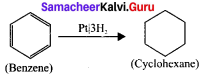Question 25.
Why cut apple turns a brown colour?

• Apples contains an enzyme called polyphenol oxidase (PPO) also known as tyrosinase.
• Cutting an apple exposes its cells to the atmospheric oxygen and oxidizes the phenolic compounds present in apples. This is called the “enzymatic browning” that turns a cut apple brown.
• In addition to apples, enzymatic browning is also evident in bananas, pears, avocados and even potatoes.

Question 26.
What are functional group inter conversions?
Organic synthesis involves functional group inter conversions. A particular functional group can be converted into other functional group by reacting it with suitable reagents. For example, the carboxylic acid group (-COOH) present in organic acids can be transformed into -COCl by treating the acid with SOCl2 reagent..

Question 27.
How will you convert alcohol into aldehyde?
When primary alcohol reacts with acidified potassium dichromate to gives aldehyde.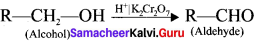Question 28.
What happen when nitrile undergoes acid hydrolysis?
When alkyl nitrile undergoes acid hydrolysis to give amide, which on further hydrolysis to give carboxylic acid.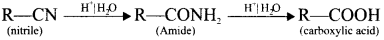Question 29.
Complete the following reactions and identify the products?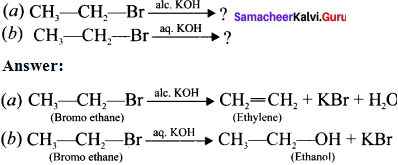Question 30.
Predict the product for the following reaction.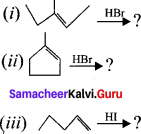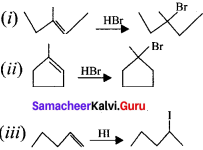Question 31.
How will you convert benzene into cyclohexane?
Benzene undergo catalytic hydrogenation in presence of platinum to give cyclohexane.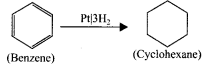Question 32.
Complete the reaction and name the product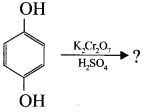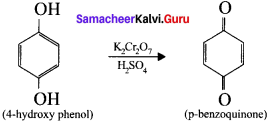Question 33.
Identify the product and mention the type of organic reaction.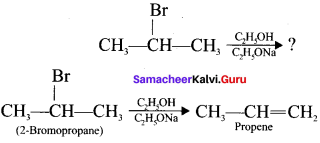This is one of the elimination reaction.

Question 34.
Complete the following reaction and identify the product.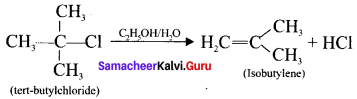Question 35.
What are substitution reaction?
In this reaction an atom or a group of atoms attached to a carbon atom is replaced by a new atom or a group of atoms.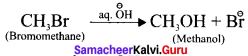Here, -Br is replaced by -OH group.

Question 36.
How will substitution reactions are classified?
Substitution reactions can be classified as,

• Nucleophilic substitution reaction.
• Electrophilic substitution reaction.

Question 37.
Draw the resonance structures for the following compounds?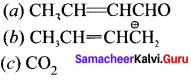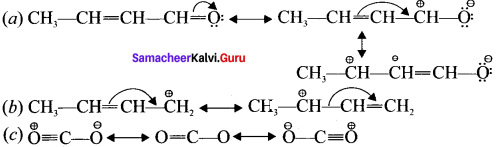Question 38.
Identify the reagents shown in box in the following equations as nucleophiles or electrophiles.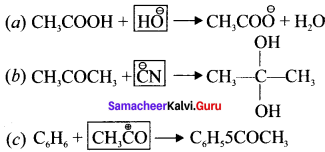In (a) $$\overset { \ominus }{ OH }$$ is nucleophile
In (b) $$\overset { \ominus }{ CN }$$ is nucleophile
In (c) CH3$$\overset { \ominus }{ CO }$$ is electrophile

Question 39.
Classify the following reactions in one of the reaction type of organic reaction.
(a) CH3CH2Br + $${ SH }^{ \ominus }$$ → CH3CH2SH + $${ Br }^{ \ominus }$$
(b) (CH3)2C = CH2 + HCI (CH3)2CCl – CH3
(c) CH3CH2Br + $${ OH }^{ \ominus }$$ → CH2 = CH2 + H2O + $${ Br }^{ \ominus }$$
(a) Nucleophilic substitution reaction.
(c) Elimination reaction.

Question 40.
Which electron displacement effect explain the following correct orders of acidity of the carboxylic acids?
CH3CH2COOH > (CH3)2 CHCOOH > (CH3)3C COOH
As the number of alkyl group increases, +I effect increases and it strengthen the O-H bond. i.e., O-H bond dissociation is very difficult.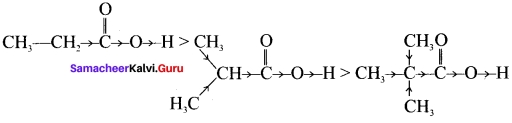Therefore +I effect explain the correct orders of acidity of the carboxylic acids.

Question 41.
Which of the two, NO2CH2CH2$${O}^{ \ominus }$$ or CH3CH2$${O}^{ \ominus }$$ is expected to be more stable and why?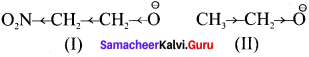(I) is more stable than (II) because NO2 group has -I effect and hence it tends to disperse the -ve charge on the 0-atom. In contrast., CH3CH, has +I effect. It therefore, tends to intensify the -ve charge and hence destabilizes it.

Samacheer Kalvi 11th Chemistry Basic Concepts of Organic Reactions 5 Marks Questions and Answers

Question 1.
Explain electron movement in organic reactions.
All organic reactions can be understood by following the electron movements.

• Lone pair becomes a bonding pair.
• Bonding pair becomes a lone pair.
• A bond breaks and becomes another bond.

The electron movement depends on the nature of the substrate, reagent and the prevailing conditions.
Type – 1.
A lone Pair to a bonding pair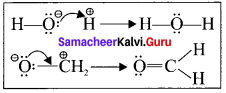Type – 2.
A bonding pair to a lone Pair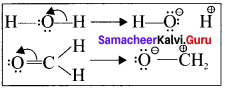Type – 3.
A bonding pair to an another bonding pair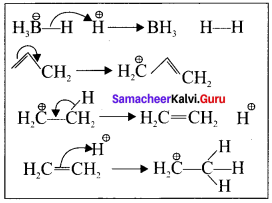Question 2.
How does inductive effect influence the reactivity and acidity of carboxylic acids?
(a) Reactivity:

• When a highly electronegative atom such as halogen is attached to a carbon then it makes the C—X bond polar.
• In such cases the -I effect of halogen facilitates the attack of an incoming nucleophile at the polarized carbon and hence increases the reactivity.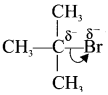• If a -I group is attacher neared to a carbonyl carbon, it decreases the availability of electron density on the carbonyl carbon and hence increases the rate of the nucleophilic addition reaction.

(b) Acidity of carboxylic acid:

• When a halogen atom is attached to the carbon which is neared to the carboxylic acid group, its -I effect withdraws the bonded electrons towards itself and makes the ionization of H+ easy.
• The acidity of various chioro acetic acid is in the following order. Cl3C-COOH > Cl2CHCOOH > ClCH2COOH The strength of the acid increases with increase in the effect of the group attached to the carboxyl group.
• Similarly the following order of acidity in the carboxylic acids is due to the +1 effect of alkyl group. (CH3)3CCOOH < (CH3)2CHCOOH <CH3COOH

Question 3.
Explain the acidic nature of phenol.

• Resonance is useful in explaining certain properties such as acidic of phenol.
• The phenoxide ion is more stabilized than phenol by resonance effect.
• The resonance favours ionisation of phenol to form H+ and shows acidity.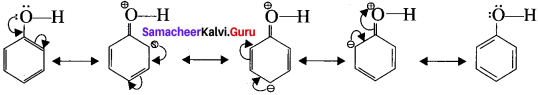phenoxide ion resonance structures.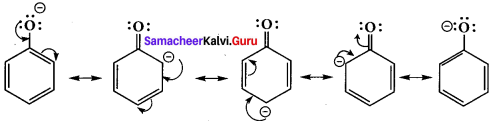• The above structures shows that there is a charge separation in the resonance structure of phenol which needs energy whereas there is no such hybrid structures in the case of phenoxide ion. This increases stability accounts for the acidic character of phenol.

Question 4.
How does hyper conjugation effect explain the stability of alkenes?

• The relative stability of various classes of carhonium ions may be explained by the number of no bond resonance structures that can be written for them.
• Such structures are arrived by shifting the bonding electrons from an adjacent C-H bond to the electron deficient carbon.
• in this way, the positive charge originally on carbon is dispersed to the hydrogen. This manner of electron release by assuming no bond character in the adjacent C-H bond is called hyper conjugation or Baker- Nathan effect.
• The greater the hyper conjugation, the greater will be the stability of the compound. The increasing order of stability can be shown as: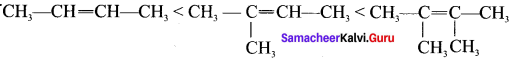• Alkyl group increases in the C=C double bond carbon, hyper conjugation increases and stability of that organic compound also increases.

Question 5.
Explain the types of addition reactions?
Addition reactions are classified into three types. They are,

An electrophilic addition reaction can be described as an addition reaction in which a reactant with multiple bonds as in a double or triple bond undergoes has it air bond broken and two new a bond are formed.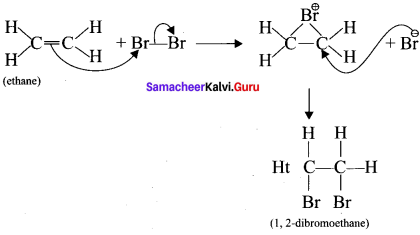A nucleophilic addition reaction is an addition reaction where a chemical compound with an electron deficient or electrophilic double or triple bond, a it bond, reacts with a nucleophilic which is an electron rich reactant with the disappearance of the double bond and creation of two new single or a bonds.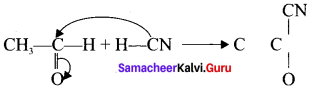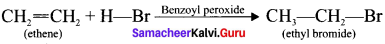Question 6.
Explain the types of substitution reaction?
Substitution reactions are classified into three types. They are,

1. Nucleophilic substitution reaction.
2. Electrophilic substitution reaction.

1. Nucleophilic substitution reaction:
A nucleophilic substitution reaction in organic chemistry is a type of reaction where a nucleophilic gets attached to the positive charged atoms or molecules of the other substance. A good example of a nucleophilic substitution reaction is the hydrolysis of alkyl bromide, under the basic conditions where in the nucleophile is nothing but the base H. whereas the leaving group is the Br°. The reaction for the following is as give below.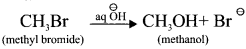2. Electrophilic substitution reaction:
The electrophilic substitution reaction involves the electrophiles. The electrophilic reactions occur mostly with the aromatic compounds. This types of substitution reaction arc basically defined as those chemical reactions where the electrophile replaces the functional group in a compound but not the hydrogen atom, sometimes hydrogen atom can be also replaced by electrophiles.
For example: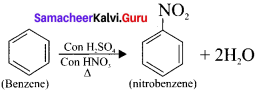A – X + Y → A – Y + X
CH4 + Cl → CH + HCl
CH3 + Cl → CH3Cl (methyl chloride)

Question 7.
For the following bond cleavages use curved-arrows to show the electron flow and classify each as homolytic or heterolytic fission. Identify reactive intermediate produced as free radical,, carbocation and carbanion?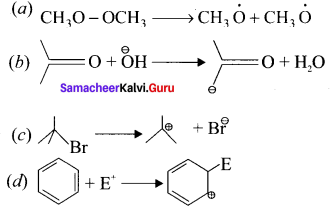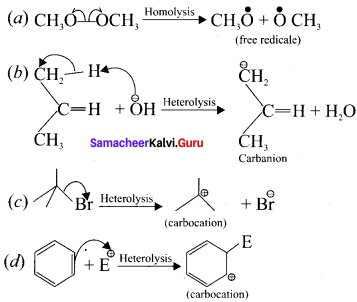Question 8.
An organic compound (A) has a molecular formula C2H6O it is one of the primary alcohol. A reacts with acidified potassium dichromate to give B. B on further undergoes to oxidation reaction to give C. C on reacts with SOCl3 to give D which ¡s chlorinated product. Identify A,B,C and D, explain with equation.
1. C2H6O is CH3-CH2-OH which is a primary alcohol (A)
2. CH3-CH2-OH (A) reacts with H+/ K2Cr2O7 to give acetaldehyde (B)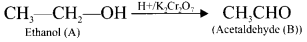3. CH3CHO (B) which on further oxidation to give acetic acid (C).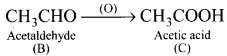4. Acetic acid reacts with SOCl2 to give acetyl chloride (D).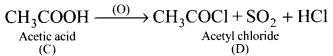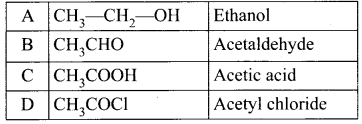Question 9.
An organic compound (A) of a molecular formula C2H4 which is a simple alkene. A reacts with dil H2S04 to give B. A again reacts with Cl2 to give C. Identify AB and C and write the equations.